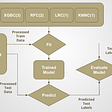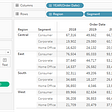# Data Analysis:

1. Importing datasets
`from sklearn import datasetsdigits = datasets.load_digits()`
`print(digits.DESCR)OUTPUT:.. _digits_dataset:Optical recognition of handwritten digits dataset--------------------------------------------------**Data Set Characteristics:**    :Number of Instances: 5620    :Number of Attributes: 64    :Attribute Information: 8x8 image of integer pixels in the range 0..16.    :Missing Attribute Values: None    :Creator: E. Alpaydin (alpaydin '@' boun.edu.tr)    :Date: July; 1998This is a copy of the test set of the UCI ML hand-written digits datasetshttps://archive.ics.uci.edu/ml/datasets/Optical+Recognition+of+Handwritten+DigitsThe data set contains images of hand-written digits: 10 classes whereeach class refers to a digit.Preprocessing programs made available by NIST were used to extractnormalized bitmaps of handwritten digits from a preprinted form. From atotal of 43 people, 30 contributed to the training set and different 13to the test set. 32x32 bitmaps are divided into nonoverlapping blocks of4x4 and the number of on pixels are counted in each block. This generatesan input matrix of 8x8 where each element is an integer in the range0..16. This reduces dimensionality and gives invariance to smalldistortions.For info on NIST preprocessing routines, see M. D. Garris, J. L. Blue, G.T. Candela, D. L. Dimmick, J. Geist, P. J. Grother, S. A. Janet, and C.L. Wilson, NIST Form-Based Handprint Recognition System, NISTIR 5469,1994... topic:: References  - C. Kaynak (1995) Methods of Combining Multiple Classifiers and Their    Applications to Handwritten Digit Recognition, MSc Thesis, Institute of    Graduate Studies in Science and Engineering, Bogazici University.  - E. Alpaydin, C. Kaynak (1998) Cascading Classifiers, Kybernetika.  - Ken Tang and Ponnuthurai N. Suganthan and Xi Yao and A. Kai Qin.    Linear dimensionalityreduction using relevance weighted LDA. School of    Electrical and Electronic Engineering Nanyang Technological University.    2005.  - Claudio Gentile. A New Approximate Maximal Margin Classification    Algorithm. NIPS. 2000.`
`digits.targetOUTPUT:array([0, 1, 2, ..., 8, 9, 8])`
`digits.data.shapeOUTPUT:(1797, 64)`
`digits.images`
`array([[ 0.,  0.,  5., 13.,  9.,  1.,  0.,  0.],       [ 0.,  0., 13., 15., 10., 15.,  5.,  0.],       [ 0.,  3., 15.,  2.,  0., 11.,  8.,  0.],       [ 0.,  4., 12.,  0.,  0.,  8.,  8.,  0.],       [ 0.,  5.,  8.,  0.,  0.,  9.,  8.,  0.],       [ 0.,  4., 11.,  0.,  1., 12.,  7.,  0.],       [ 0.,  2., 14.,  5., 10., 12.,  0.,  0.],       [ 0.,  0.,  6., 13., 10.,  0.,  0.,  0.]])`
• Import pyplot module which is under matplotlib as plt.
• The imshow() function is used to display data as an image; i.e. on a 2D regular raster.
• cmap = gray_r displays a grayscale image.
• interpolation= ‘nearest’ displays an image without trying to interpolate between pixels if the display resolution is not the same as the image resolution.
• The title() function is used to display the title on the graph.
`import matplotlib.pyplot as pltplt.imshow(digits.images, cmap=plt.cm.gray_r, interpolation='nearest')plt.title('Visualizing array')#save the figureplt.savefig('plot2.png', dpi=100, bbox_inches='tight')`
• The figure() function in the pyplot module of the matplotlib library is used to create a new figure with a specified size of (15,4).
• subplots_adjust(hspace=0.8) is used to adjust the space between the rows of the subplots.
• Combine two lists using the zip() function for easier handling inside the plotting loop.
• enumerate() method adds a counter to an iterable and returns it. The returned object is a enumerate object.
• subplot() function is used to add a subplot to a current figure at the specified grid position.
`import numpy as npplt.figure(figsize=(15,4))plt.subplots_adjust(hspace=0.8)for index, (image, label) in enumerate(zip(digits.data[0:10], digits.target[0:10])):plt.subplot(2, 5, index+1)plt.imshow(np.reshape(image, (8,8)), cmap=plt.cm.gray)plt.title('Training: %i\n' % label, fontsize =12)#save the figureplt.savefig('plot1.png', dpi=300, bbox_inches='tight')`
`digits.target.size`
`1797`
`# flatten the imagesn_samples = len(digits.images)data = digits.images.reshape((n_samples, -1))`
`from sklearn.model_selection import train_test_splitx_train, x_test, y_train, y_test = train_test_split(data, digits.target, test_size=0.2, random_state=0)`

# 11 .Support Vector Classifier

`from sklearn import svmsvc = svm.SVC(gamma=0.001, C=100.)`

## Train the model

`svc.fit(x_train, y_train)OUTPUT:SVC(C=100.0, break_ties=False, cache_size=200, class_weight=None, coef0=0.0,    decision_function_shape='ovr', degree=3, gamma=0.001, kernel='rbf',    max_iter=-1, probability=False, random_state=None, shrinking=True,    tol=0.001, verbose=False)`

## Test the model

`y_pred = svc.predict(x_test)`
`_, axes = plt.subplots(nrows=1, ncols=4, figsize=(10, 3))for ax, image, prediction in zip(axes, x_test, y_pred):ax.set_axis_off()image = image.reshape(8, 8)ax.imshow(image, cmap=plt.cm.gray_r, interpolation='nearest')ax.set_title(f'Prediction: {prediction}')# save the figureplt.savefig('plot7.png', dpi=300, bbox_inches='tight')`
`score = svc.score(x_test, y_test)print('Accuracy Score: {0}'.format(score))OUTPUT:Accuracy Score: 0.9916666666666667`
`from sklearn.metrics import confusion_matrix,classification_report,accuracy_scoreimport seaborn as snsimport pandas as pdlabels=['0','1','2', '3','4','5','6','7','8','9']f, ax = plt.subplots(figsize=(10,10))cm=confusion_matrix(y_test,y_pred)sns.heatmap(cm, annot=True,ax=ax,cmap="Dark2_r")#labels, title and ticksax.set_xlabel('Predicted labels')ax.set_ylabel('True labels')ax.set_title('Accuracy Score: {0} \n Confusion Matrix'.format(np.round(score,2)))ax.xaxis.set_ticklabels(labels)ax.yaxis.set_ticklabels(labels)plt.savefig('plot3.png', dpi=300, bbox_inches='tight')plt.show()f, ax = plt.subplots(figsize=(6,6))class_report=classification_report(y_test,y_pred,target_names=labels, output_dict=True)sns.heatmap(pd.DataFrame(class_report).iloc[:-1, :].T, annot=True,ax=ax,cmap="Dark2_r")ax.set_title('Classification Report')plt.savefig('plot4.png', dpi=300, bbox_inches='tight')plt.show()`
`from sklearn.linear_model import LogisticRegressionlogisticRegr = LogisticRegression()import warningswarnings.filterwarnings("ignore")logisticRegr.fit(x_train, y_train)OUTPUT:LogisticRegression(C=1.0, class_weight=None, dual=False, fit_intercept=True,                   intercept_scaling=1, l1_ratio=None, max_iter=100,                   multi_class='auto', n_jobs=None, penalty='l2',                   random_state=None, solver='lbfgs', tol=0.0001, verbose=0,                   warm_start=False)`
`y_pred=logisticRegr.predict(x_test)`
`# Use score method to get accuracy of modelscore = logisticRegr.score(x_test, y_test)print('Accuracy Score: {0}'.format(score))OUTPUT:Accuracy Score: 0.9666666666666667`
`​from sklearn.metrics import confusion_matrix,classification_report,accuracy_scoreimport seaborn as snsimport pandas as pdlabels=['0','1','2', '3','4','5','6','7','8','9']f, ax = plt.subplots(figsize=(10,10))cm=confusion_matrix(y_test,y_pred)sns.heatmap(cm, annot=True,ax=ax,cmap="Dark2_r")#labels, title and ticksax.set_xlabel('Predicted labels')ax.set_ylabel('True labels')ax.set_title('Accuracy Score: {0} \n Confusion Matrix'.format(np.round(score,2)))ax.xaxis.set_ticklabels(labels)ax.yaxis.set_ticklabels(labels)plt.savefig('plot5.png', dpi=300, bbox_inches='tight')plt.show()f, ax = plt.subplots(figsize=(6,6))class_report=classification_report(y_test,y_pred,target_names=labels, output_dict=True)sns.heatmap(pd.DataFrame(class_report).iloc[:-1, :].T, annot=True,ax=ax,cmap="Dark2_r")ax.set_title('Classification Report')plt.savefig('plot6.png', dpi=300, bbox_inches='tight')plt.show()`
`from sklearn.tree import DecisionTreeClassifierdt = DecisionTreeClassifier(criterion = 'gini')`
`​dt.fit(x_train, y_train)`
`DecisionTreeClassifier(ccp_alpha=0.0, class_weight=None, criterion='gini',                       max_depth=None, max_features=None, max_leaf_nodes=None,                       min_impurity_decrease=0.0, min_impurity_split=None,                       min_samples_leaf=1, min_samples_split=2,                       min_weight_fraction_leaf=0.0, presort='deprecated',                       random_state=None, splitter='best')`
`y_pred=dt.predict(x_test)`
`# Use score method to get accuracy of modelscore = dt.score(x_test, y_test)print('Accuracy Score: {0}'.format(score))OUTPUT:Accuracy Score: 0.8583333333333333`

# Confusion matrix and Classification report of the model

`​from sklearn.metrics import confusion_matrix,classification_report,accuracy_scoreimport seaborn as snsimport pandas as pdlabels=['0','1','2', '3','4','5','6','7','8','9']f, ax = plt.subplots(figsize=(10,10))cm=confusion_matrix(y_test,y_pred)sns.heatmap(cm, annot=True,ax=ax,cmap="Dark2_r")#labels, title and ticksax.set_xlabel('Predicted labels')ax.set_ylabel('True labels')ax.set_title('Accuracy Score: {0} \n Confusion Matrix'.format(np.round(score,2)))ax.xaxis.set_ticklabels(labels)ax.yaxis.set_ticklabels(labels)plt.savefig('plot7.png', dpi=300, bbox_inches='tight')plt.show()f, ax = plt.subplots(figsize=(6,6))class_report=classification_report(y_test,y_pred,target_names=labels, output_dict=True)sns.heatmap(pd.DataFrame(class_report).iloc[:-1, :].T, annot=True,ax=ax,cmap="Dark2_r")ax.set_title('Classification Report')plt.savefig('plot8.png', dpi=300, bbox_inches='tight')plt.show()`
`from sklearn.ensemble import RandomForestClassifierrc = RandomForestClassifier(n_estimators = 150)`

## Train the model

`rc.fit(x_train, y_train)`
`RandomForestClassifier(bootstrap=True, ccp_alpha=0.0, class_weight=None,                       criterion='gini', max_depth=None, max_features='auto',                       max_leaf_nodes=None, max_samples=None,                       min_impurity_decrease=0.0, min_impurity_split=None,                       min_samples_leaf=1, min_samples_split=2,                       min_weight_fraction_leaf=0.0, n_estimators=150,                       n_jobs=None, oob_score=False, random_state=None,                       verbose=0, warm_start=False)`
`y_pred=rc.predict(x_test)`
`# Use score method to get accuracy of modelscore = rc.score(x_test, y_test)print('Accuracy Score: {0}'.format(score))OUTPUT:Accuracy Score: 0.975`

# Confusion matrix and Classification report of the model

`from sklearn.metrics import confusion_matrix,classification_report,accuracy_scoreimport seaborn as snsimport pandas as pdlabels=['0','1','2', '3','4','5','6','7','8','9']f, ax = plt.subplots(figsize=(10,10))cm=confusion_matrix(y_test,y_pred)sns.heatmap(cm, annot=True,ax=ax,cmap="Dark2_r")#labels, title and ticksax.set_xlabel('Predicted labels')ax.set_ylabel('True labels')ax.set_title('Accuracy Score: {0} \n Confusion Matrix'.format(np.round(score,2)))ax.xaxis.set_ticklabels(labels)ax.yaxis.set_ticklabels(labels)plt.savefig('plot9.png', dpi=300, bbox_inches='tight')plt.show()f, ax = plt.subplots(figsize=(6,6))class_report=classification_report(y_test,y_pred,target_names=labels, output_dict=True)sns.heatmap(pd.DataFrame(class_report).iloc[:-1, :].T, annot=True,ax=ax,cmap="Dark2_r")ax.set_title('Classification Report')plt.savefig('plot10.png', dpi=300, bbox_inches='tight')plt.show()`

# Conclusion:

## More from Neha Kumari

Love podcasts or audiobooks? Learn on the go with our new app.

## US Road Accidents Data Analysis## Analysis和Analytics的中文翻譯都是”分析”，但意思大不同 / What is the difference between Analysis and Analytics## Build a LDA model for classification with Gensim## Best Python Books for Beginners## Understanding a Data Science Project## ‘We Rate Dogs’ : Twitter Data Analysis## Better Data Equals Better Healthcare## [Data Science] Journey to Summer 2021 Internship## Credit Card Default Detection — Kaggle## What is Data Blending in Tableau?## What does this additional term in the linear regression equation mean?## How to handle missing data (in different scenarios)